WEBINAR
Magnetic Levitation for Energy Harvesting
Thursday, June 22, 2023
Time
SESSION 1
SESSION 2
CEST (GMT +2)
03:00 PM
08:00 PM
EDT (GMT -4)
09:00 AM
02:00 PM
HOME / Applications / Electromechanical FEM simulation of a T-Shaped Electromagnetic Actuator using EMS for SOLIDWORKS

# Electromechanical FEM simulation of a T-Shaped Electromagnetic Actuator using EMS for SOLIDWORKS

View 3D results Used Tools:## Problem description

3D FEM simulation of a T-Shaped electromagnetic actuator was performed, and the results are discussed in this article. Analyzing DC actuator helps to predict and evaluate magnetic quantities and parameters (magnetic flux, magnetic force, speed, positions, etc.) which are used in the efficient design of an actuator. EMS for SOLIDWORKS software is used to study the proposed electromagnet . The force produced by the coil on the moving part is calculated versus ampere-turns and air gap distance. The obtained results were compared to experimental measurement.  Motion analysis is coupled to EMS analysis in order to compute linear displacement and velocity of the actuator.

Figure 1 shows the geometry of the simulated electromagnetic actuator while table 1 contains the main dimensions . Figure 2 illustrates the 3D model built inside SOLIDWORKS.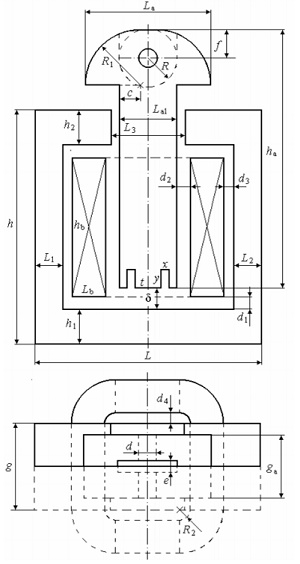Figure 1 - Geometry of the T-shaped electromagnet 

Table 1 - Dimensions of the electromagnet
 h 52.5 g 19.8 f 6.3 hb 31.2 h1 7.9 ha 57.8 R 6.5 Lb 7.5 h2 7.9 La 28.3 R1 12.3 d1 2.4 L 50.9 La1 13 ga 14.3 d2 3 L1 6.35 c 4.65 x 1.6 d3 2.1 L2 6.35 d 4 y 4.2 d4 2.25 L3 16.5 e 2.6 t 6 R2 2.4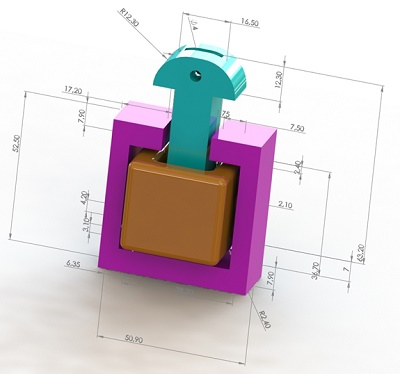Figure 1 - 3D model of the electromagnet structure

## 3D EM simulation using EMS inside SOLIDWORKS

Parametric Analysis using EMSEM simulation helps to study the different aspects of the electromagnet covering several scenarios. EMS allows to run multiple study cases into a single analysis by enabling parameterization of both simulation and geometrical variables. The Magnetostatic study type of EMS is used to compute and visualize the magnetic results such as magnetic flux density, magnetic field intensity, force, torque, etc. In this article, the magnetic force generated by the actuator is computed versus both air gap distance and ampere-turns.

Simulation setup1. The simulation is started by creating a parameterized Magnetostatic analysis where the ampere-turns and the air gap distance are the parametric variables. Table 2 shows the simulated scenarios.

Table 2 - Simulation scenarios

 Scenarios Air gap length (mm) Ampere-turns (A-t) scenario 1 2 575 scenario 2 3 575 scenario 3 4 575 scenario 4 5 575 scenario 5 6 575 scenario 6 7 575 scenario 7 2 460 scenario 8 3 460 scenario 9 4 460 scenario 10 5 460 scenario 11 6 460 scenario 12 7 460 scenario 13 2 345 scenario 14 3 345 scenario 15 4 345 scenario 16 5 345 scenario 17 6 345 scenario 18 7 345

2. The ferromagnetic parts are made of non-oriented steel. Figure 3 contains the BH curve of the used steel. The coil is made of copper.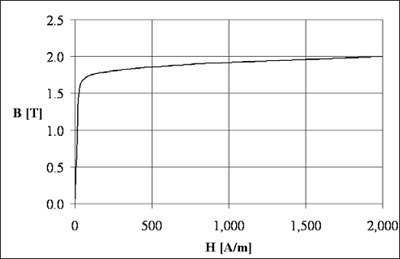Figure 3 - B-H curve 

3. Figure below shows the meshed model. It is possible to define specific mesh element size on selected surfaces or bodies.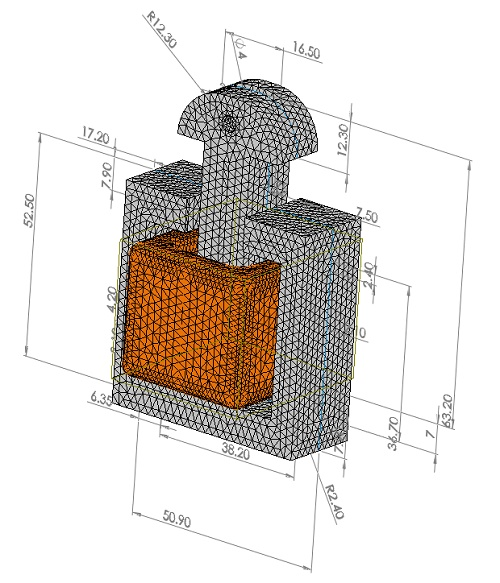Figure 4 - Meshed model

Results and discussionFor the case of 460 A-t and 6 mm air gap, the magnetic flux density is plotted in Figure 5. The magnetic flux is following the steel path where the permeability is high. Figure 6 shows a vector plot of the magnetic flux density when the ampere-turns is 575 A-t.
The magnetic force computed by EMS and measured by experimental tests ref  is shown in Figures 7. This force which is produced by the coil and evaluated on the moving plunger of the T-shaped electromagnet, is directly proportional to the coil ampere-turns. It is about 6.3 N for 575 A-t compared to 4 N for 460 A-t. Unlike the ampere-turns, the force becomes higher with smaller air gap distance. Larger air gap occurs to a larger magnetic reluctance and consequently smaller magnetic flux and force.

The force curves have a maximum value at the 2 mm and a minimum value at 7 mm of air gap length for each curve.  The highest force is measured for 575 A-t and 2 mm air gap length (see Figure 7).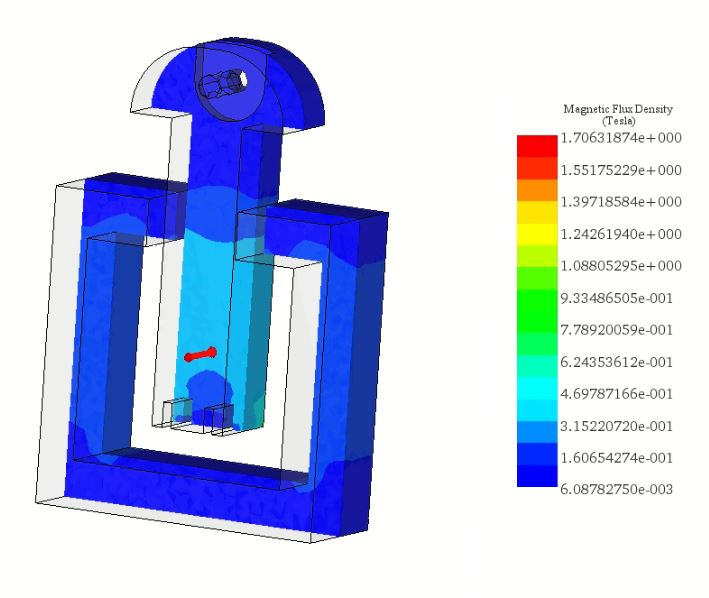Figure 5 - Cross section plot of the magnetic flux density (460A-t, 6mm)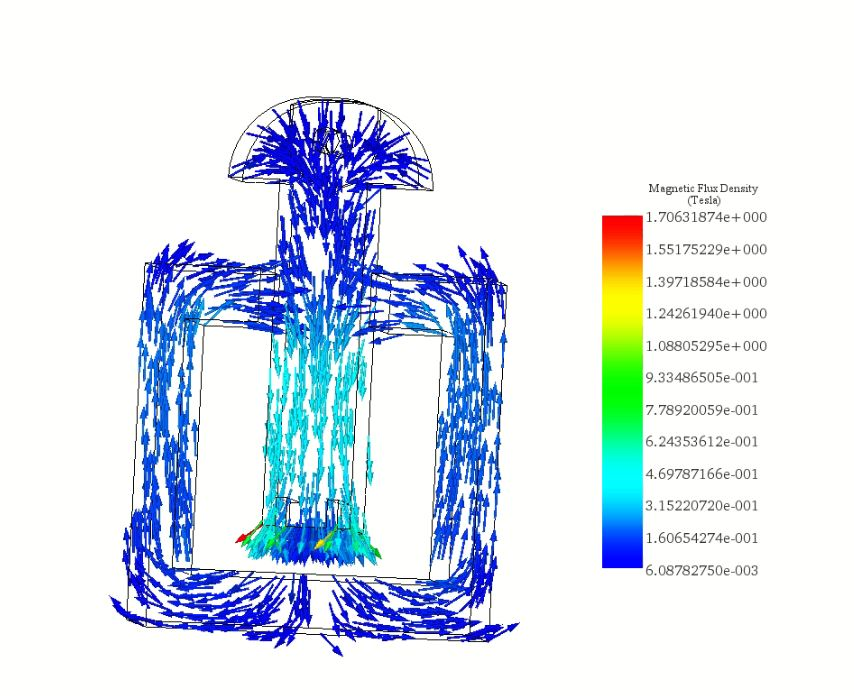Figure 6 - Vector plot of the magnetic flux densityFigure 7 - Comparison of the magnetic force computed by EMS and measured by experimental tests

## Electromagnetic simulation coupled to SOLIDWORKS Motion

EMS has the capability to couple electromagnetic fields with motion simulation inside SOLIDWORKS. EMS computes the electromagnetic force and feeds it to SW Motion. The solver of SW Motion uses this force and converts it into a mechanical motion. Figure 8 shows SW motion study settings.

Figure 9 contains a cross section view of the magnetic flux density plot in the moving plunger and the core at 0.025s (AT=575 A-t). At t=0s, the position of the plunger is Y=0 mm (air gap distance is 7 mm).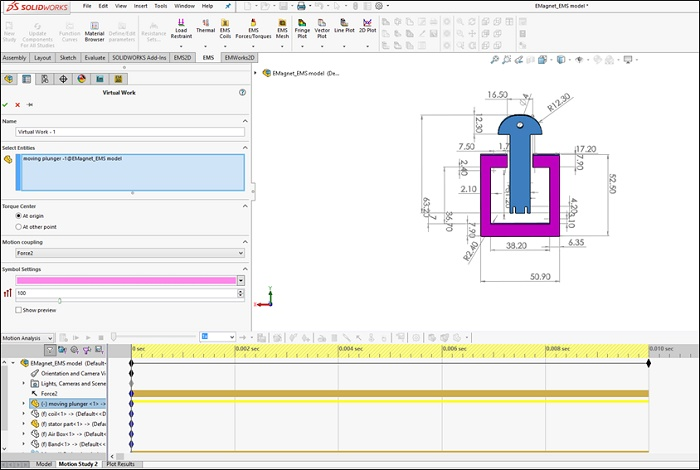Figure 8 - Motion study settings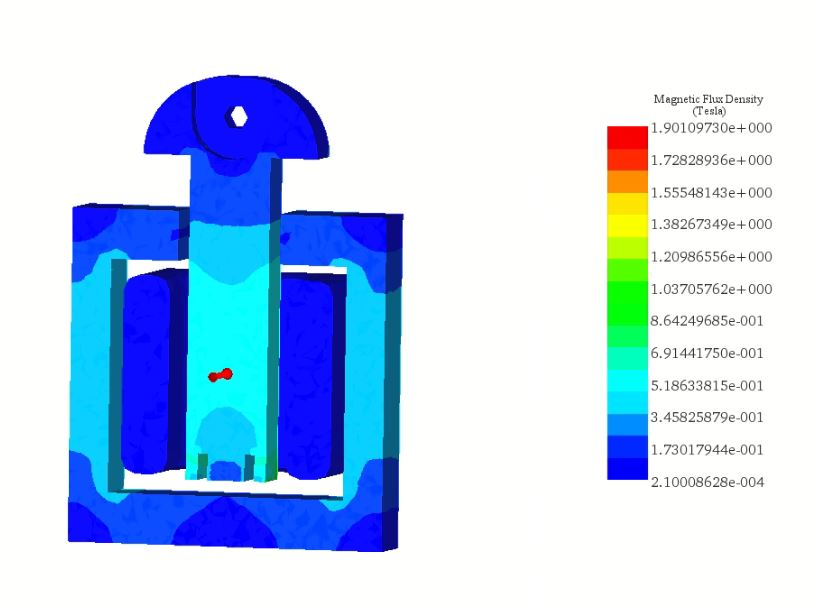Figure 9 - Section plot of the magnetic flux density

Figures 10 a) and 10b) show the magnetic force computed by EMS on the moving part and referred to the SW motion analysis, respectively versus position and time. At 0 mm, the force is equal to F=1.4N while it is about F=6.20N at Y= -0.0049m (see Figure 10a). These results are in good agreement with the results published in Figure 7 (575 A-t). The force on the plunger is 33.16N at 0.01s (see Figure 10b).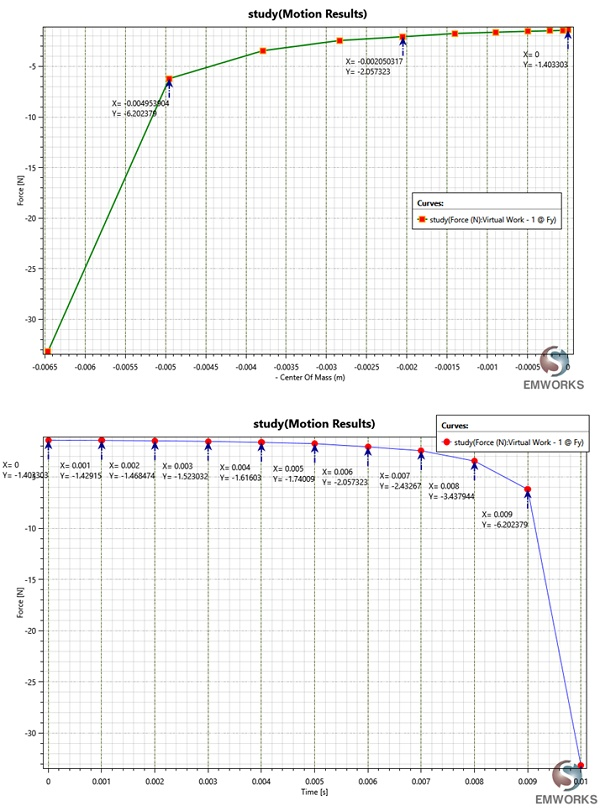Figure 10 - Force results, a) versus position, b) versus time

Figures 11a) and 11b) show respectively the position of the plunger versus time and the instantaneous linear velocity. The velocity reached its maximum value 1.67 m/s at 0.01s. It increases with the force.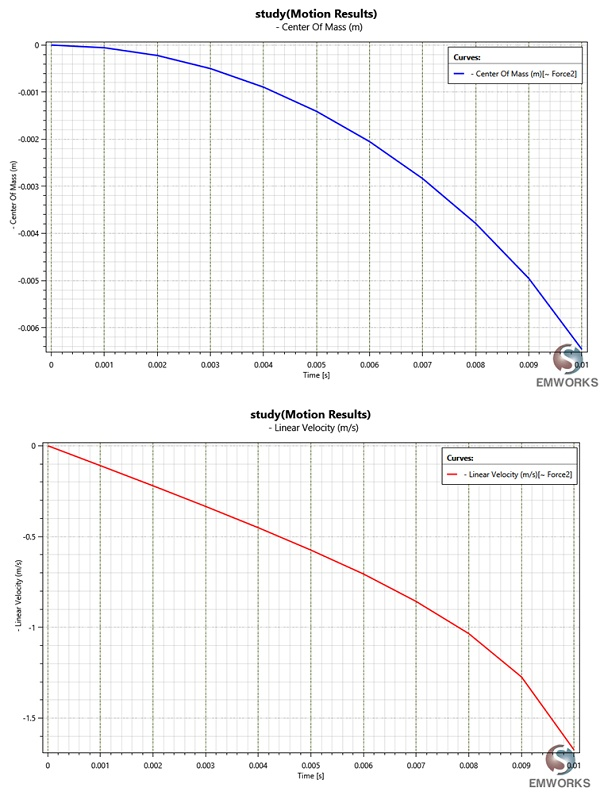Figure 11 - a) Center of mass of the plunger versus time, b) Linear velocity of the plunger

## Conclusion

Electromagnetic actuators are widely used in several applications. Designing innovative types of electromagnet is becoming possible thanks to 3D CAD and simulation. It helps to estimate the magnetic flux in different components and regions and the generated magnetic force on the moving plunger. Evaluating the magnetic forces is needed to design additional components like springs which ensure the falling back of the plunger. It also helps to compute the linear displacement and velocity of the actuator. EMS allows to parameterize geometrical and simulation variables and run multi-scenarios in a single simulation. The coupling of electromagnetic study of EMS with motion analysis of SOLIDWORKS provide additional and useful information about position and speed of the moving plunger.

#### References

: Alin-Iulian DOLAN, Ivan YATCHEV and Krastio HINOV . COMPARISON OF DIFFERENT FORMULATIONS AND TECHNIQUES
FOR 3D STATIC FORCE COMPUTATION OF A T-SHAPED ELECTROMAGNET.

View 3D model and 3D results